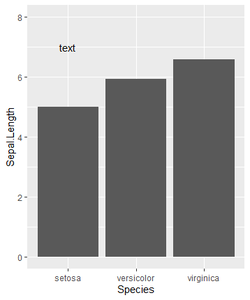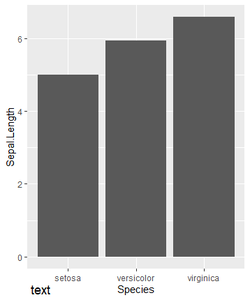Related Articles
• Explore Our Geeks Community
• Data visualization with R and ggplot2

# Annotate Text Outside of ggplot2 Plot in R

Ggplot2 is based on the grammar of graphics, the idea that you can build every graph from the same few components: a data set, a set of geoms—visual marks that represent data points, and a coordinate system. There are many scenarios where we need to annotate outside the plot area or specific area as per client requirements. In this case, the ggplot2 library comes very handy with its sub-options to get the required output and with good customization options for data visualizations.

To add annotations in R using ggplot2, annotate() function is used.

Syntax: annotate()

Parameters:

• geom : specify text
• x : x axis location
• y : y axis location
• label : custom textual content
• color : color of textual content
• size : size of text
• fontface : fontface of text
• angle : angle of text

### Approach

• Import library
• Create a normal plot
• Add annotate() function with required parameters

Let us first see how annotations are added inside the plot, so that the difference in position of the annotations can be understood better.

Examples :

## R

 `library``(ggplot2) ` ` `  `Dt = iris ` ` `  `ggplot``(Dt,``aes``(x=Species,y=Sepal.Length)) +   ` `geom_bar``(stat = ``"summary"``, fun = ``"mean"``) +   ` `annotate``(``"text"``, x = 1, y = 7, label = ``"Arbitrary text"``) +  ` `coord_cartesian``(ylim = ``c``(0, 8), clip = ``"off"``)`

Output:Now let us visualize with annotations outside the plot.

Example:

## R

 `library``(ggplot2) ` ` `  `Dt = iris ` ` `  `ggplot``(Dt,``aes``(x=Species,y=Sepal.Length)) +   ` `geom_bar``(stat = ``"summary"``, fun = ``"mean"``) +   ` `annotate``(``"text"``, x = 1, y = -1, label = ``"text"``) +   ` `coord_cartesian``(ylim = ``c``(0, 8), clip = ``"off"``)`

Output:Whether you're preparing for your first job interview or aiming to upskill in this ever-evolving tech landscape, GeeksforGeeks Courses are your key to success. We provide top-quality content at affordable prices, all geared towards accelerating your growth in a time-bound manner. Join the millions we've already empowered, and we're here to do the same for you. Don't miss out - check it out now!

Previous
Next